### IMO Shortlist 2005 problem G6

Kvaliteta:
Avg: 0,0
Težina:
Avg: 8,0
Let$ABC$ be a triangle, and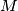$M$ the midpoint of its side$BC$. Let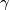$\gamma$ be the incircle of triangle$ABC$. The median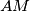$AM$ of triangle$ABC$ intersects the incircle$\gamma$ at two points$K$ and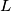$L$. Let the lines passing through$K$ and$L$, parallel to$BC$, intersect the incircle$\gamma$ again in two points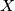$X$ and$Y$. Let the lines$AX$ and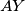$AY$ intersect$BC$ again at the points$P$ and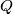$Q$. Prove that$BP = CQ$.
Izvor: Međunarodna matematička olimpijada, shortlist 2005